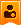# 人民广场至高铁北站公交车运行调整时间公告

[tr]  [td=30]

[/td]  [td=55]

[/td]  [td=105]

[/td]  [td=55]

[/td]  [td=55]

[/td]  [td=416]

[tr]  [td=30]
1
[/td]  [td=55]
D8392
[/td]  [td=105]

[/td]  [td=55]
7:48
[/td]  [td=55]
7:51
[/td]  [td=416]

[tr]  [td=30]
2
[/td]  [td=55]
D3784
[/td]  [td=105]

[/td]  [td=55]
8:20
[/td]  [td=55]
8:22
[/td]  [td=416]

[tr]  [td=30]
3
[/td]  [td=55]
D3937
[/td]  [td=105]

[/td]  [td=55]
9:04
[/td]  [td=55]
9:08
[/td]  [td=416]

[tr]  [td=30]
4
[/td]  [td=55]
D8462
[/td]  [td=105]

[/td]  [td=55]
9:18
[/td]  [td=55]
9:20
[/td]  [td=416]

[tr]  [td=30]
5
[/td]  [td=55]
D3792
[/td]  [td=105]

[/td]  [td=55]
9:56
[/td]  [td=55]
9:58
[/td]  [td=416]

[tr]  [td=30]
6
[/td]  [td=55]
D3923
[/td]  [td=105]

[/td]  [td=55]
10:35
[/td]  [td=55]
10:39
[/td]  [td=416]

[tr]  [td=30]
7
[/td]  [td=55]
D3808
[/td]  [td=105]

[/td]  [td=55]
11:00
[/td]  [td=55]
11:02
[/td]  [td=416]

[tr]  [td=30]
8
[/td]  [td=55]
D3903
[/td]  [td=105]

[/td]  [td=55]
11:18
[/td]  [td=55]
11:22
[/td]  [td=416]

[tr]  [td=30]
9
[/td]  [td=55]
D3902
[/td]  [td=105]

[/td]  [td=55]
11:27
[/td]  [td=55]
11:29
[/td]  [td=416]

[tr]  [td=30]
10
[/td]  [td=55]
D8461
[/td]  [td=105]

[/td]  [td=55]
11:55
[/td]  [td=55]
11:59
[/td]  [td=416]

[tr]  [td=30]
11
[/td]  [td=55]
D3807
[/td]  [td=105]

[/td]  [td=55]
12:10
[/td]  [td=55]
12:14
[/td]  [td=416]

[tr]  [td=30]
12
[/td]  [td=55]
D3816
[/td]  [td=105]

[/td]  [td=55]
12:33
[/td]  [td=55]
12:35
[/td]  [td=416]

[tr]  [td=30]
13
[/td]  [td=55]
D3811
[/td]  [td=105]

[/td]  [td=55]
12:37
[/td]  [td=55]
12:41
[/td]  [td=416]

[tr]  [td=30]
14
[/td]  [td=55]
D3904
[/td]  [td=105]

[/td]  [td=55]
12:58
[/td]  [td=55]
13:00
[/td]  [td=416]

[tr]  [td=30]
15
[/td]  [td=55]
D3953
[/td]  [td=105]

[/td]  [td=55]
12:58
[/td]  [td=55]
13:02
[/td]  [td=416]

[tr]  [td=30]
16
[/td]  [td=55]
D3820
[/td]  [td=105]

[/td]  [td=55]
13:10
[/td]  [td=55]
13:12
[/td]  [td=416]

[tr]  [td=30]
17
[/td]  [td=55]
D8464
[/td]  [td=105]

[/td]  [td=55]
13:25
[/td]  [td=55]
13:27
[/td]  [td=416]

[tr]  [td=30]
18
[/td]  [td=55]
D3819
[/td]  [td=105]

[/td]  [td=55]
13:29
[/td]  [td=55]
13:33
[/td]  [td=416]

[tr]  [td=30]
19
[/td]  [td=55]
D3961
[/td]  [td=105]

[/td]  [td=55]
14:00
[/td]  [td=55]
14:04
[/td]  [td=416]

[tr]  [td=30]
20
[/td]  [td=55]
D8391
[/td]  [td=105]

[/td]  [td=55]
14:29
[/td]  [td=55]
14:33
[/td]  [td=416]

[tr]  [td=30]
21
[/td]  [td=55]
D3934
[/td]  [td=105]

[/td]  [td=55]
15:05
[/td]  [td=55]
15:07
[/td]  [td=416]

[tr]  [td=30]
22
[/td]  [td=55]
D3831
[/td]  [td=105]

[/td]  [td=55]
15:18
[/td]  [td=55]
15:22
[/td]  [td=416]

[tr]  [td=30]
23
[/td]  [td=55]
D3839
[/td]  [td=105]

[/td]  [td=55]
15:46
[/td]  [td=55]
15:50
[/td]  [td=416]

[tr]  [td=30]
24
[/td]  [td=55]
D8398
[/td]  [td=105]

[/td]  [td=55]
15:58
[/td]  [td=55]
16:00
[/td]  [td=416]

[tr]  [td=30]
25
[/td]  [td=55]
D3847
[/td]  [td=105]

[/td]  [td=55]
16:44
[/td]  [td=55]
16:48
[/td]  [td=416]

[tr]  [td=30]
26
[/td]  [td=55]
D3927
[/td]  [td=105]

[/td]  [td=55]
17:04
[/td]  [td=55]
17:08
[/td]  [td=416]

[tr]  [td=30]
27
[/td]  [td=55]
D3851
[/td]  [td=105]

[/td]  [td=55]
17:19
[/td]  [td=55]
17:23
[/td]  [td=416]

[tr]  [td=30]
28
[/td]  [td=55]
D3950
[/td]  [td=105]

[/td]  [td=55]
17:46
[/td]  [td=55]
17:48
[/td]  [td=416]

[tr]  [td=30]
29
[/td]  [td=55]
D3855
[/td]  [td=105]

[/td]  [td=55]
17:47
[/td]  [td=55]
17:51
[/td]  [td=416]

[tr]  [td=30]
30
[/td]  [td=55]
D8363
[/td]  [td=105]

[/td]  [td=55]
18:36
[/td]  [td=55]
18:40
[/td]  [td=416]

[tr]  [td=30]
31
[/td]  [td=55]
D3859
[/td]  [td=105]

[/td]  [td=55]
19:05
[/td]  [td=55]
19:09
[/td]  [td=416]

[tr]  [td=30]
32
[/td]  [td=55]
D3928
[/td]  [td=105]

[/td]  [td=55]
19:16
[/td]  [td=55]
19:18
[/td]  [td=416]

[tr]  [td=30]
33
[/td]  [td=55]
D3958
[/td]  [td=105]

[/td]  [td=55]
19:46
[/td]  [td=55]
19:48
[/td]  [td=416]

[tr]  [td=30]
34
[/td]  [td=55]
D3791
[/td]  [td=105]

[/td]  [td=55]
19:52
[/td]  [td=55]
19:56
[/td]  [td=416]

[tr]  [td=30]
35
[/td]  [td=55]
D8255
[/td]  [td=105]

[/td]  [td=55]
20:05
[/td]  [td=55]
20:09
[/td]  [td=416]

[tr]  [td=30]
36
[/td]  [td=55]
D8366
[/td]  [td=105]

[/td]  [td=55]
20:32
[/td]  [td=55]
20:34
[/td]  [td=416]

[tr]  [td=30]
37
[/td]  [td=55]
D3962
[/td]  [td=105]

[/td]  [td=55]
21:00
[/td]  [td=55]
21:02
[/td]  [td=416]

[tr]  [td=30]
38
[/td]  [td=55]
D8463
[/td]  [td=105]

[/td]  [td=55]
21:59
[/td]  [td=55]
22:03
[/td]  [td=416]主题 积分

838

1#发表于  2019-07-10 12:17:25
[tr]  [td=2,1,320]

[/td]  [td=121]

[/td]  [td=121]

[/td]  [td=121]

[/td]  [td=121]

[/td]  [td=122]

[tr]  [td=1,2,121]

[/td]  [td=198]

[/td]  [td=121]
6:50
[/td]  [td=121]
10:15
[/td]  [td=121]
12:35
[/td]  [td=121]
15:00
[/td]  [td=122]
[tr]  [td=198]

[/td]  [td=121]
8:25
[/td]  [td=121]
11:05
[/td]  [td=121]
13:35
[/td]  [td=121]
16:00
[/td]  [td=122]
[tr]  [td=1,2,121]

[/td]  [td=198]

[/td]  [td=121]
7:20
[/td]  [td=121]
10:30
[/td]  [td=121]
13:10
[/td]  [td=121]
15:35
[/td]  [td=122]
17:50
[tr]  [td=198]

[/td]  [td=121]
9:10
[/td]  [td=121]
11:30
[/td]  [td=121]
14:10
[/td]  [td=121]
16:30
[/td]  [td=122]
18:40
[tr]  [td=1,2,121]

[/td]  [td=198]

[/td]  [td=121]
8:20
[/td]  [td=121]
11:20
[/td]  [td=121]
13:45
[/td]  [td=121]
16:00
[/td]  [td=122]
18:20
[tr]  [td=198]

[/td]  [td=121]
9:55
[/td]  [td=121]
12:20
[/td]  [td=121]
14:35
[/td]  [td=121]
17:10
[/td]  [td=122]
19:20
[tr]  [td=1,2,121]

[/td]  [td=198]

[/td]  [td=121]
9:00
[/td]  [td=121]
11:40
[/td]  [td=121]
14:20
[/td]  [td=121]
16:50
[/td]  [td=122]
[tr]  [td=198]

[/td]  [td=121]
10:20
[/td]  [td=121]
12:45
[/td]  [td=121]
15:10
[/td]  [td=121]
17:50
[/td]  [td=122]
[tr]  [td=1,2,121]

[/td]  [td=198]

[/td]  [td=121]
9:35
[/td]  [td=121]
12:10
[/td]  [td=121]
14:40
[/td]  [td=121]
17:10
[/td]  [td=122]
[tr]  [td=198]

[/td]  [td=121]
10:40
[/td]  [td=121]
13:15
[/td]  [td=121]
15:25
[/td]  [td=121]
17:55
[/td]  [td=122]
[tr]  [td=1,2,121]

[/td]  [td=198]

[/td]  [td=121]
17:00
[/td]  [td=121]
18:50
[/td]  [td=121]
21:10
[/td]  [td=121]
[/td]  [td=122]
[tr]  [td=198]

[/td]  [td=121]
17:40
[/td]  [td=121]
20:10
[/td]  [td=121]
22:10
[/td]  [td=121]
[/td]  [td=122]
[tr]  [td=7,1,927]
说明 :1.途径站点：县人民广场→商都后门→农业局→金穗农贸市场→国土局→石化生活区→百采厂大门→上法街路口→汽车总站→炼油厂路口→jj大道路口→jj义圩路口→超大物流→jj科园路口→jj产业园大门→石化廉租房→那桑屯→子桑安置点→田东北站  。2.票价:3元/ 人次  。3.发班时间：黑色标示时间为人民广场发班时间，红色标示时间为田东北站发班时间。4.本表根据动车到达时间动态作相应调整发班时间，同时视客源增加情况，随时增加车辆班次。
 人民广场至高铁北站公交车运行调整时间公告 自7月10日起，结合田东北站高铁发车时刻变化情况，我公司18路公交线（人民广场至高铁北站）运行时间也相应做出如下调整，现公告如下： [tr]  [td=7,1,927]  田东县兴隆公交公司18路公交车（人民广场至高铁北站）发车时间表
广西田东县兴隆公共汽车运输有限责任公司

[tr]  [td=6,1,716]
2019.7.10田东北站动车时刻表主题 积分

22844

2#发表于  2019-07-10 13:23:51
 已收藏，谢谢楼主主题 积分

13018

3#发表于  2019-07-10 14:53:20
 应按从广场发车的时间顺序来排序更容易看明白，至于有多少辆公交车，每辆车走几趟对大多数旅客来说并不重要主题 积分

458

4#发表于  2019-07-10 16:50:44
 乱七八糟的，看都看不懂主题 积分

46582

5#发表于  2019-07-10 19:08:24
 你可以装路路通或12306。主题 积分

1138

6#发表于  2019-07-10 19:17:55
 乱主题 积分

46582

7#发表于  2019-07-11 09:51:18
 装路路通或12306，详细过。主题 积分

3605

8#发表于  2019-07-11 14:43:48
 .发班时间：黑色标示时间为人民广场发班时间，红色标示时间为田东北站发班时间。。。哪里有红色？主题 积分

59847

9#发表于  2019-07-12 16:00:31
 {:24:}主题 积分

4012

10#发表于  2019-07-12 23:30:15
 希望能准点按所标的路线发车主题 积分

355

11#发表于  2019-07-19 11:18:37
 上星期天，试着按图标的时间17点50分在广场等公车，结果车到了但直接被忽略，门都没开直直开走，弄得一同等车去火车站的五六个外地客人面面相觑，无言以对。{:14:}主题 积分

718

12#发表于  2019-07-22 21:53:22
 写明始发班时间和到终点站时间就可以了，你写每一台车的轮次乱七八糟的，旅客根本不需要。主题 积分

718

13#发表于  2019-07-22 21:55:11
 列成表格不是看得清楚过吗？主题 积分

45

14#发表于  2019-07-22 22:10:44
 {:26:}{:26:}{:26:}目前我还没坐过田东的高铁主题 积分

150

15#发表于  2019-07-26 16:09:10
 {:5:}{:5:}本主题已关闭，不再接受新内容!